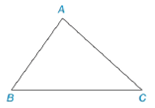Chapter 7.1, Problem 47EElementary Geometry For College St...

7th Edition
Alexander + 2 others
ISBN: 9781337614085

Solutions

Chapter
SectionElementary Geometry For College St...

7th Edition
Alexander + 2 others
ISBN: 9781337614085
Textbook Problem

In Exercises 39 and 42, refer to the line segments shown.Use the following theorem to construct a triangle similar to the given triangle but with sides that are twice the length of those of the given triangle.Theorem: If the lengths of the three pairs of sides for two triangles are in proportion, then those triangles are similar (SSS˜).To determine

To construct:

The triangle similar to the given triangle but with sides that are twice the length of those of the given triangle.

Explanation

Theorem:

If the lengths of the three pairs of sides for two triangles are in proportion, then those triangles are similar.

Calculation:

Construct the given triangle ABC as shown in figure.

To construct a triangle similar to ABC but twice the size, follow the below procedure:

1. Take any arbitrary point Y and draw a horizontal line XZ equal to twice the length of BC.

2. Take Y as center and draw an arc equal to twice the length of AB above the line YZ and take Z as center and draw an arc equal to twice the length of AC above the line YZ to obtain the intersection point X.

3. Draw the lines XY and XZ to obtain the triangle twice the size of the triangle ABC as shown below:

Still sussing out bartleby?

Check out a sample textbook solution.

See a sample solution

The Solution to Your Study Problems

Bartleby provides explanations to thousands of textbook problems written by our experts, many with advanced degrees!

Get Started

Convert the expressions in Exercises 6584 to power form. x2y

Finite Mathematics and Applied Calculus (MindTap Course List)

Multiply: 6.121.32

Elementary Technical Mathematics

Find for defined implicity by .

Study Guide for Stewart's Multivariable Calculus, 8th

The graph of f(x) = 3x5 − 5x3 is:

Study Guide for Stewart's Single Variable Calculus: Early Transcendentals, 8th